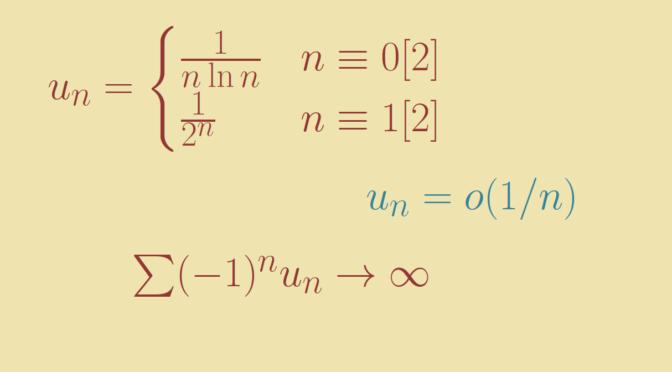Counterexamples around series (part 2)

We follow the article counterexamples around series (part 1) providing additional funny series examples.

If $$\sum u_n$$ converges and $$(u_n)$$ is non-increasing then $$u_n = o(1/n)$$?

This is true. Let’s prove it.
The hypotheses imply that $$(u_n)$$ converges to zero. Therefore $$u_n \ge 0$$ for all $$n \in \mathbb N$$. As $$\sum u_n$$ converges we have $\displaystyle \lim\limits_{n \to \infty} \sum_{k=n/2}^{n} u_k = 0.$ Hence for $$\epsilon \gt 0$$, one can find $$N \in \mathbb N$$ such that $\epsilon \ge \sum_{k=n/2}^{n} u_k \ge \frac{1}{2} (n u_n) \ge 0$ for all $$n \ge N$$. Which concludes the proof.

$$\sum u_n$$ convergent is equivalent to $$\sum u_{2n}$$ and $$\sum u_{2n+1}$$ convergent?

Is not true as we can see taking $$u_n = \frac{(-1)^n}{n}$$. $$\sum u_n$$ converges according to the alternating series test. However for $$n \in \mathbb N$$ $\sum_{k=1}^n u_{2k} = \sum_{k=1}^n \frac{1}{2k} = 1/2 \sum_{k=1}^n \frac{1}{k}.$ Hence $$\sum u_{2n}$$ diverges as the harmonic series diverges.

$$\sum u_n$$ absolutely convergent is equivalent to $$\sum u_{2n}$$ and $$\sum u_{2n+1}$$ absolutely convergent?

This is true and the proof is left to the reader.

$$\sum u_n$$ is a positive convergent series then $$(\sqrt[n]{u_n})$$ is bounded?

Is true. If not, there would be a subsequence $$(u_{\phi(n)})$$ such that $$\sqrt[\phi(n)]{u_{\phi(n)}} \ge 2$$. Which means $$u_{\phi(n)} \ge 2^{\phi(n)}$$ for all $$n \in \mathbb N$$ and implies that the sequence $$(u_n)$$ is unbounded. In contradiction with the convergence of the series $$\sum u_n$$.

If $$(u_n)$$ is strictly positive with $$u_n = o(1/n)$$ then $$\sum (-1)^n u_n$$ converges?

It does not hold as we can see with $u_n=\begin{cases} \frac{1}{n \ln n} & n \equiv 0  \\ \frac{1}{2^n} & n \equiv 1  \end{cases}$ Then for $$n \in \mathbb N$$ $\sum_{k=1}^{2n} (-1)^k u_k \ge \sum_{k=1}^n \frac{1}{2k \ln 2k} – \sum_{k=1}^{2n} \frac{1}{2^k} \ge \sum_{k=1}^n \frac{1}{2k \ln 2k} – 1.$ As $$\sum \frac{1}{2k \ln 2k}$$ diverges as can be proven using the integral test with the function $$x \mapsto \frac{1}{2x \ln 2x}$$, $$\sum (-1)^n u_n$$ also diverges.